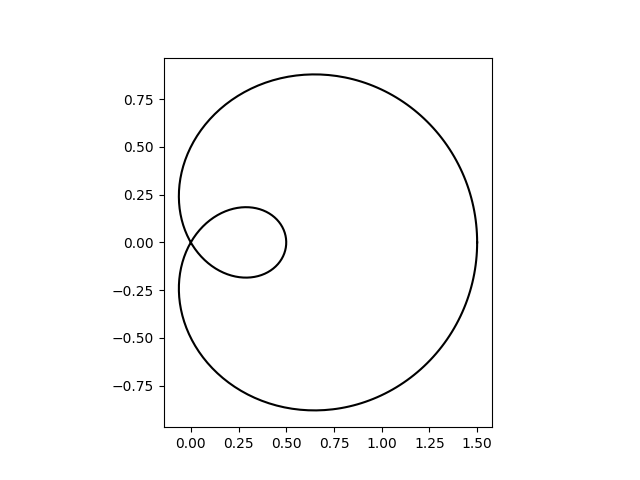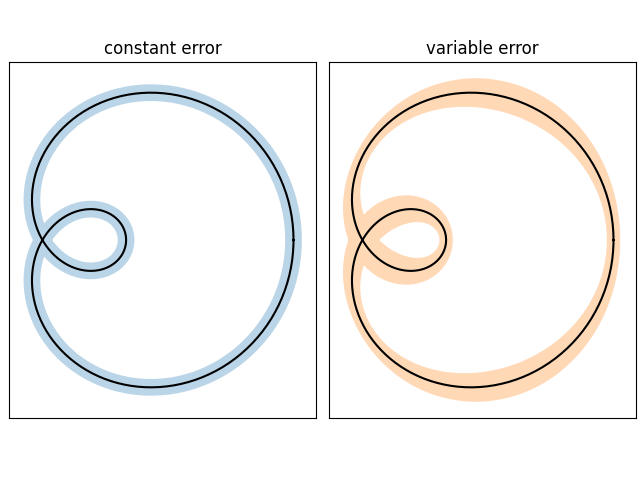# Curve with error band#

This example illustrates how to draw an error band around a parametrized curve.

A parametrized curve x(t), y(t) can directly be drawn using plot.

import numpy as np

import matplotlib.pyplot as plt
from matplotlib.path import Path
from matplotlib.patches import PathPatch

N = 400
t = np.linspace(0, 2 * np.pi, N)
r = 0.5 + np.cos(t)
x, y = r * np.cos(t), r * np.sin(t)

fig, ax = plt.subplots()
ax.plot(x, y, "k")
ax.set(aspect=1)[None]


An error band can be used to indicate the uncertainty of the curve. In this example we assume that the error can be given as a scalar err that describes the uncertainty perpendicular to the curve in every point.

We visualize this error as a colored band around the path using a PathPatch. The patch is created from two path segments (xp, yp), and (xn, yn) that are shifted by +/- err perpendicular to the curve (x, y).

Note: This method of using a PathPatch is suited to arbitrary curves in 2D. If you just have a standard y-vs.-x plot, you can use the simpler fill_between method (see also Filling the area between lines).

def draw_error_band(ax, x, y, err, **kwargs):
# Calculate normals via centered finite differences (except the first point
# which uses a forward difference and the last point which uses a backward
# difference).
dx = np.concatenate([[x - x], x[2:] - x[:-2], [x[-1] - x[-2]]])
dy = np.concatenate([[y - y], y[2:] - y[:-2], [y[-1] - y[-2]]])
l = np.hypot(dx, dy)
nx = dy / l
ny = -dx / l

# end points of errors
xp = x + nx * err
yp = y + ny * err
xn = x - nx * err
yn = y - ny * err

vertices = np.block([[xp, xn[::-1]],
[yp, yn[::-1]]]).T
codes = np.full(len(vertices), Path.LINETO)
codes = codes[len(xp)] = Path.MOVETO
path = Path(vertices, codes)

axs = (plt.figure(constrained_layout=True)
.subplots(1, 2, sharex=True, sharey=True))
errs = [
(axs, "constant error", 0.05),
(axs, "variable error", 0.05 * np.sin(2 * t) ** 2 + 0.04),
]
for i, (ax, title, err) in enumerate(errs):
ax.set(title=title, aspect=1, xticks=[], yticks=[])
ax.plot(x, y, "k")
draw_error_band(ax, x, y, err=err,
facecolor=f"C{i}", edgecolor="none", alpha=.3)

plt.show()References

The use of the following functions, methods, classes and modules is shown in this example:

Keywords: matplotlib code example, codex, python plot, pyplot Gallery generated by Sphinx-Gallery